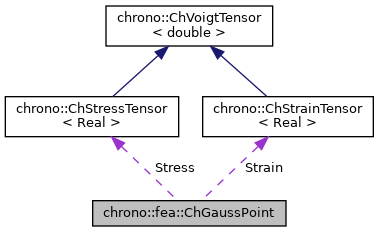chrono::fea::ChGaussPoint Class Reference

Description

Class for a Gauss point, that has a position (1D-3D) and a weight.

It also contains the strain and the stress tensor.

#include <ChGaussPoint.h>

Collaboration diagram for chrono::fea::ChGaussPoint:[legend]

Public Member Functions

ChGaussPoint (int number, ChVector<> *coord, double weight)
Create a Gauss point with given number, coordinates and weight.

ChVector GetLocalCoordinates () const
Return local coordinates.

void SetLocalCoordinates (const ChVector<> &c)

ChVector GetCoordinates () const
Return absolute coordinates.

void SetCoordinates (const ChVector<> &c)
Set absolute coordinates.

double GetWeight () const

void SetWeight (double w)

int GetNumber () const
Return number of the point.

Public Attributes

ChMatrixDynamicMatrB
Matrix of partial derivatives: to obtain strain & stress.

ChStrainTensor Strain
Strain tensor.

ChStressTensor Stress
Stress tensor.

The documentation for this class was generated from the following files:
• /builds/uwsbel/chrono/src/chrono/fea/ChGaussPoint.h
• /builds/uwsbel/chrono/src/chrono/fea/ChGaussPoint.cpp